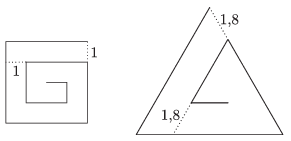Mathematical and Physical Journal
for High Schools
Issued by the MATFUND Foundation
 Already signed up? New to KöMaL?

#Problem C. 1305. (September 2015)

C. 1305. A spiral is made out of a square of side $\displaystyle n$ units, as shown in the figure, by always breaking the line 1 unit before reaching the already existing part of the spiral. Similarly, a spiral is made out of a regular triangle of side $\displaystyle 1.8n$ units by breaking the line 1.8 units before closing up. (The diagram shows the two spirals for the case of $\displaystyle n = 4$.) For what value of n will the lengths of the two spirals be equal?(5 pont)

Deadline expired on October 12, 2015.

### Statistics:

 250 students sent a solution. 5 points: 102 students. 4 points: 61 students. 3 points: 10 students. 2 points: 9 students. 1 point: 5 students. 0 point: 58 students. Unfair, not evaluated: 5 solutionss.

Problems in Mathematics of KöMaL, September 2015